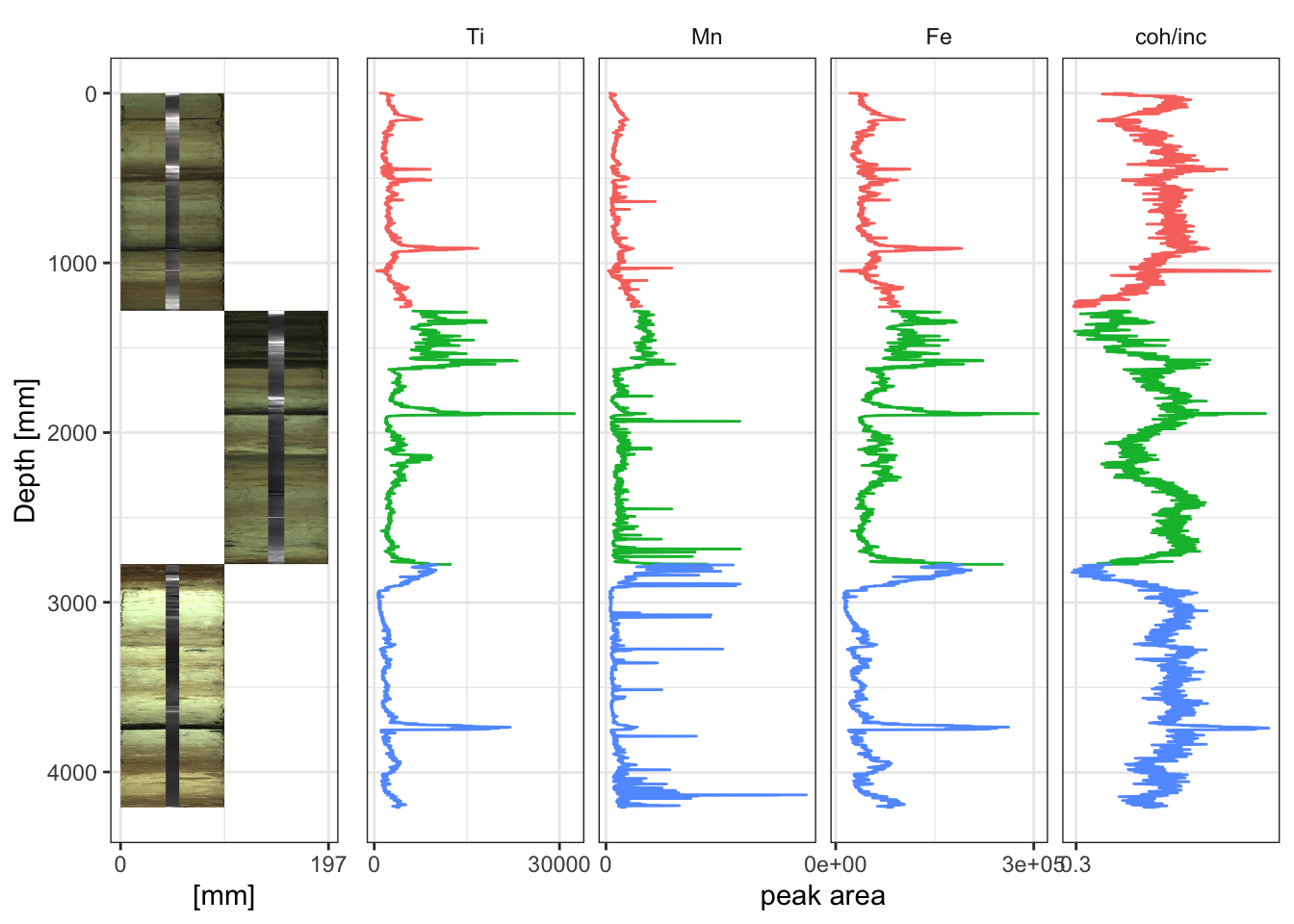## 4.3 Combining Images with XRF Data

It is possible to combine different plots. For example, it is often desirable to plot the XRF data alongside the core imagery. By not passing `coord_fixed(ratio = 1)` to the image plot, its relative width can be controlled via `widths =`, which makes for a visually pleasing plot. Note also that the y-axis labels have been removed from all but one of the plots to avoid duplication.

``````egg::ggarrange(imagePlot + theme_paleo(),
xrfStrat + theme(axis.title.y = element_blank(),
axis.text.y  = element_blank(),
axis.ticks.y = element_blank()),
ncol = 2,
widths = c(1, 4) # these are relative. For c(1, 5), the first plot will be 1/5th the width of the second.
)``````